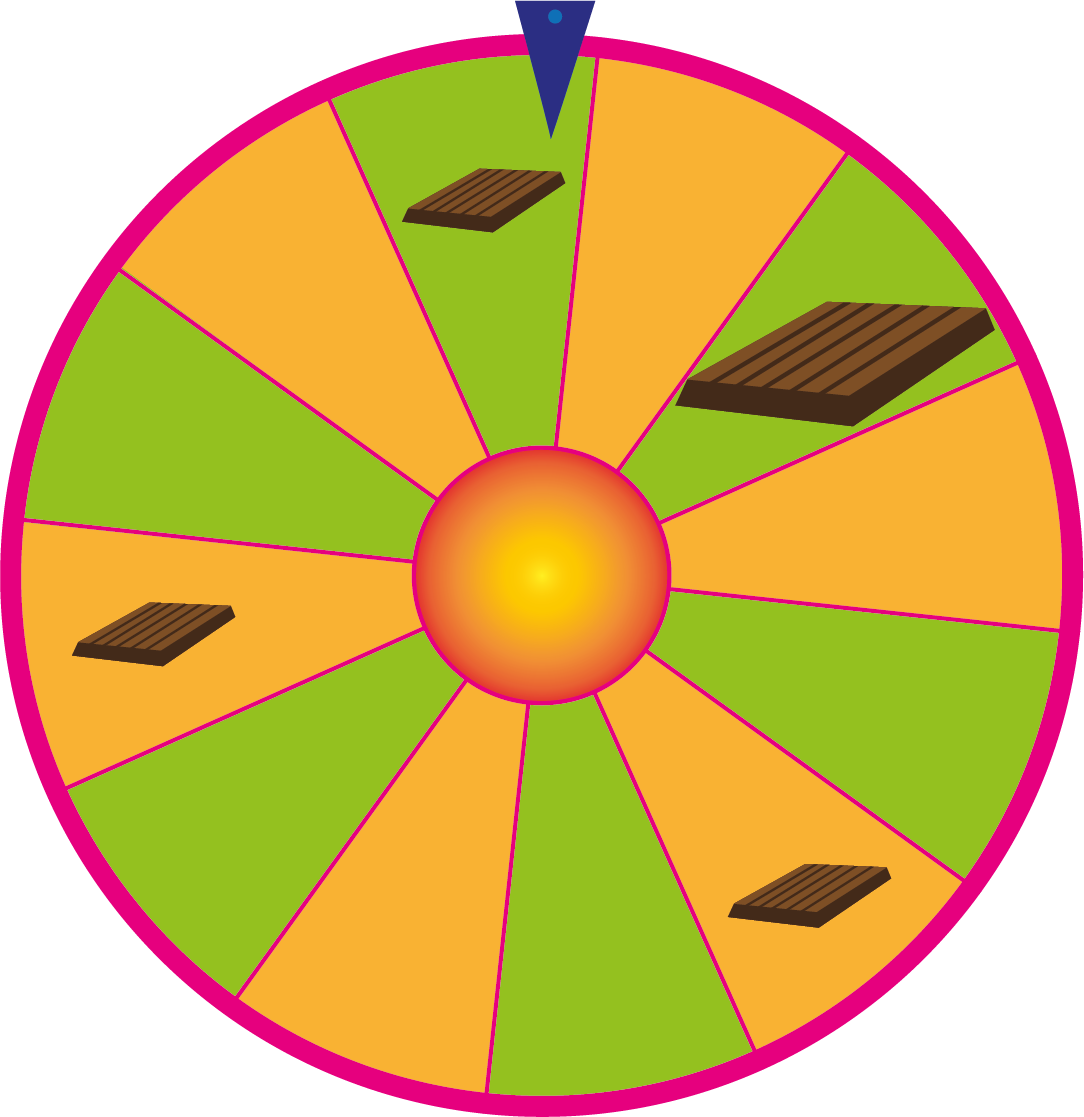# How Probability Is Calculated

Imagine you are at the state fair or at an amusement park, and that you to play the wheel of fortune. You can win small and large chocolate prizes.The wheel starts to spin. There’s only one field on the wheel that wins you the biggest chocolate prize. In total, there are 12 different fields on the wheel that can stop under the pin. What’s the probability that you win the biggest chocolate?

Probabilities are often written as fractions. You must therefore find out what should be in the numerator, and what should be in the denominator. The numerator states the number of possibilities that will win you the biggest chocolate. In this case, there’s only one place the wheel can stop at to give you the biggest prize. Therefore, it should say 1 in the numerator. The denominator states the total number of outcomes, which in this case is the number of fields that can stop under the pin. There are 12 different possibilities, so it must say 12 in the denominator. The probability that you win the biggest chocolate is therefore $\frac{1}{12}$.

Rule

When we are absolutely sure that something will happen, we say the probability is equal to 1, which is the same as $100$ %.

When we are absolutely sure that something will not happen, we say the probability is equal to 0, which is the same as $0$ %.

The probability of something happening is between 0 and 1, as in the case with the prize wheel. The closer to 1 the probability is, the more likely the event is to occur.

The denominator must state how many possible outcomes there are. There are still 12 different fields that can stop under the pin. Therefore, it should say 12 in the denominator. The probability of winning a chocolate prize (small or large), is then $\frac{4}{12}=\frac{1}{3}$.